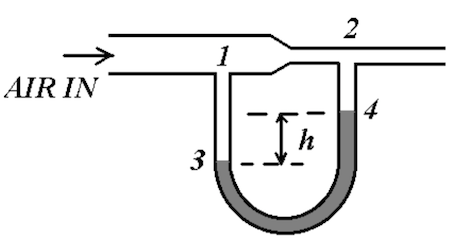Problem: The Venturi meter is used to measure the speed of air (density ρa) and is shown in the figure below. It consists of a horizontal pipe with variable sectional areas, and a flexible hose, partially filled with water (density ρw), connecting two points in the pipe with different areas. Air enters the meter at speed v1 and atmospheric pressure po. The sectional area of the meter is A1 at point 1 and A2 at point 2.a) What is the speed of the air at point 2?b) What is the pressure of the air at point 2?c) What is the value of h, the difference between the water levels at 3 and 4?d) If the speed v1 doubles, how much bigger (or smaller) does h become?Notice that h is not a given parameter of the problem.

FREE Expert Solution
96% (479 ratings)
Problem Details

The Venturi meter is used to measure the speed of air (density ρa) and is shown in the figure below. It consists of a horizontal pipe with variable sectional areas, and a flexible hose, partially filled with water (density ρw), connecting two points in the pipe with different areas. Air enters the meter at speed v1 and atmospheric pressure po. The sectional area of the meter is A1 at point 1 and A2 at point 2.

a) What is the speed of the air at point 2?

b) What is the pressure of the air at point 2?

c) What is the value of h, the difference between the water levels at 3 and 4?

d) If the speed v1 doubles, how much bigger (or smaller) does h become?

Notice that h is not a given parameter of the problem.